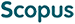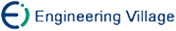Qin Hong, Wang Hao, Wei Xiaochao, Zheng Zhihua. Secure Constant-Round Multi-User k-Means Clustering Protocol[J]. Journal of Computer Research and Development, 2020, 57(10): 2188-2200. doi: 10.7544/issn1000-1239.2020.20200407
 Citation: Qin Hong, Wang Hao, Wei Xiaochao, Zheng Zhihua. Secure Constant-Round Multi-User k-Means Clustering Protocol[J]. Journal of Computer Research and Development, 2020, 57(10): 2188-2200. doi: 10.7544/issn1000-1239.2020.20200407# Secure Constant-Round Multi-User k-Means Clustering Protocol• In most of the practical applications of cluster calculation, the data usually comes from different users, and the cluster algorithm often needs to be calculated on the joint data of them. For privacy protection purposes, users do not want to share their private data with others. Therefore, how to implement multi-user cluster calculation in a privacy-preserving way has attracted widespread attention. In this paper, we research the secure computation of k-means cluster algorithm in the scenario where multiple users hold data, and design a constant round secure multi-user k-means cluster protocol. In this protocol, users encrypt data using additive homomorphic encryption and upload data to an independent assisted server. Then, this server implements the secure computation of multiplication and Euclidean distance by interacting with cluster calculator holding the private key. In addition, we also design a minimal element marking protocol and a division protocol for homomorphic ciphertext based on the ABY framework. The protocol realizes the conversion among homomorphic ciphertexts, arithmetic shares and Yao shares through constant round interaction without expensive bit-decomposition technology. We give the formal security proof of main protocol and all sub-protocols in the semi-honest model. Finally, we give the efficiency analysis and performance test of our scheme.
•/DownLoad:  Full-Size Img  PowerPoint